# GloVe vectors for sentiment analysis¶

Last time, we discuss how GloVe vectors are trained for sentiment analysis.

We will use a package called torchtext, which works with torch, to explore and use GloVe vectors

In :
import csv
import torch
import torch.nn as nn
import torch.nn.functional as F
import torch.optim as optim
import torchtext
import numpy as np
import matplotlib.pyplot as plt


## GloVe vectors¶

We will use the 6B version of the GloVe vector. There are several versions of the embedding that is available. We will start with the smallest one, which is the 50 dimensional vector. Later on, we will use the 100 dimensional word vectors.

In :
# The first time you run this will download a ~823MB file
glove = torchtext.vocab.GloVe(name="6B", dim=50, max_vectors=20000)


Let's look at what the embedding of the word "car" looks like:

In :
glove['car']

Out:
tensor([ 0.4769, -0.0846,  1.4641,  0.0470,  0.1469,  0.5082, -1.2228, -0.2261,
0.1931, -0.2976,  0.2060, -0.7128, -1.6288,  0.1710,  0.7480, -0.0619,
-0.6577,  1.3786, -0.6804, -1.7551,  0.5832,  0.2516, -1.2114,  0.8134,
0.0948, -1.6819, -0.6450,  0.6322,  1.1211,  0.1611,  2.5379,  0.2485,
-0.2682,  0.3282,  1.2916,  0.2355,  0.6147, -0.1344, -0.1324,  0.2740,
-0.1182,  0.1354,  0.0743, -0.6195,  0.4547, -0.3032, -0.2188, -0.5605,
1.1177, -0.3659])

It is troch tensor with dimension (50,). It is difficult to determine what each number in this embedding means, if anything. However, we know that there is structure in this embedding space. That is, distances in this embedding space is meaningful.

So, let's compute the Euclidean distance between (the embedding of) the word "car" and several other words. The Euclidean distance (or the $L_2$ norm of the distance vector) is computed as $\sqrt{\sum_i (x_i - y_i)^2}$ for word vectors $x$ and $y$.

In :
word = 'car'
other = ['bike', 'girl', 'computer', 'space', 'was', 'kite']
for w in other:
dist = torch.norm(glove[word] - glove[w]) # euclidean distnace
print(w, float(dist))

bike 4.049488544464111
girl 5.822113513946533
computer 5.773685932159424
space 5.68005895614624
was 4.979914665222168
kite 6.30803108215332


The Euclidean distance is a distance measure, and the smaller the distance, the closer the embeddings are to each other. The word "bike" is closest to the word "car" out of all the words in that list.

Instead of using the Euclidean distance, we can use a different distance measure. For example, we can compute the cosine similarity, a measure of the angle between the two vectors:

In :
for w in other:
dist = torch.nn.functional.cosine_similarity(glove['car'].unsqueeze(0), glove[w].unsqueeze(0))
print(w, float(dist))

bike 0.7256852388381958
girl 0.477798193693161
computer 0.5121089816093445
space 0.47960782051086426
was 0.5771499276161194
kite 0.1811121255159378


The cosine similiarity is a similarity measure, and the larger the similarity, the "closer" the word embeddings are to each other. The word "bike" is still closest to the word "car" out of all the words in that list using this measure.

We can compare other pairs of words in the same way:

In :
word = 'candy'
other = ['chocolate', 'sugar', 'cartoon', 'computer', 'bike', 'girl', 'was', 'car']
for w in other:
dist = torch.norm(glove[word] - glove[w])
cosdist = torch.nn.functional.cosine_similarity(glove[word].unsqueeze(0), glove[w].unsqueeze(0))
print(w, float(dist), float(cosdist), sep="\t")

chocolate	3.198564052581787	0.8237079977989197
sugar	4.297327041625977	0.6400755643844604
cartoon	5.009539604187012	0.4607061445713043
computer	6.3510918617248535	0.2876129150390625
bike	5.718624114990234	0.2939280569553375
girl	5.41274881362915	0.4525235891342163
was	5.975126266479492	0.21566349267959595
car	5.906295299530029	0.35387226939201355

In :
word = 'happy'
other = ['good', 'sad', 'tired', 'bad', 'unhappy', 'cry']
for w in other:
dist = torch.norm(glove[word] - glove[w])
cosdist = torch.nn.functional.cosine_similarity(glove[word].unsqueeze(0), glove[w].unsqueeze(0))
print(w, float(dist), float(cosdist), sep="\t")

good	2.7146358489990234	0.8574146628379822
tired	3.142784357070923	0.7785122394561768
unhappy	3.2546586990356445	0.7167829275131226
cry	3.326601266860962	0.7269507050514221


The second example is interesting, because "happy" and "sad" represent human sentiments that are the opposite ends of the sentiment spectrum. For more examples, see https://lamyiowce.github.io/word2viz/

## Sentiment Analysis¶

Sentiment Analysis is the problem of identifying the writer's sentiment given a piece of text. It can be applied to movie reviews, feedback of other forms, emails, tweets, and even course evaluations.

Rudimentary forms of sentiment analysis might involve scoring each word on a scale from "sad" to "happy", then averaging the "happiness score" of each word in a piece of text. This technique has obvious drawbacks: it won't be able to handle negation, sarcasm, or any complex syntactical form. We can do better. In fact, we will use the sentiment analysis task as an example in the next few lectures.

Specifically, we will use the Sentiment140 data set. This dataset contains tweets containing either a positive or negative emoticon. Our goal is to determine whether which type of emoticon the tweet (with the emoticon removed) contained. THe dataset was actually collected by a group of students, just like you, who are doing their first machine learning project.

You can download the data here: http://help.sentiment140.com/for-students

Let's look at the data:

In :
import csv

def get_data():
return csv.reader(open("training.1600000.processed.noemoticon.csv", "rt", encoding="latin-1"))

for i, line in enumerate(get_data()):
if i > 10:
break
print(line)

['0', '1467810369', 'Mon Apr 06 22:19:45 PDT 2009', 'NO_QUERY', '_TheSpecialOne_', "@switchfoot http://twitpic.com/2y1zl - Awww, that's a bummer.  You shoulda got David Carr of Third Day to do it. ;D"]
['0', '1467810672', 'Mon Apr 06 22:19:49 PDT 2009', 'NO_QUERY', 'scotthamilton', "is upset that he can't update his Facebook by texting it... and might cry as a result  School today also. Blah!"]
['0', '1467810917', 'Mon Apr 06 22:19:53 PDT 2009', 'NO_QUERY', 'mattycus', '@Kenichan I dived many times for the ball. Managed to save 50%  The rest go out of bounds']
['0', '1467811184', 'Mon Apr 06 22:19:57 PDT 2009', 'NO_QUERY', 'ElleCTF', 'my whole body feels itchy and like its on fire ']
['0', '1467811193', 'Mon Apr 06 22:19:57 PDT 2009', 'NO_QUERY', 'Karoli', "@nationwideclass no, it's not behaving at all. i'm mad. why am i here? because I can't see you all over there. "]
['0', '1467811372', 'Mon Apr 06 22:20:00 PDT 2009', 'NO_QUERY', 'joy_wolf', '@Kwesidei not the whole crew ']
['0', '1467811592', 'Mon Apr 06 22:20:03 PDT 2009', 'NO_QUERY', 'mybirch', 'Need a hug ']
['0', '1467811594', 'Mon Apr 06 22:20:03 PDT 2009', 'NO_QUERY', 'coZZ', "@LOLTrish hey  long time no see! Yes.. Rains a bit ,only a bit  LOL , I'm fine thanks , how's you ?"]
['0', '1467811795', 'Mon Apr 06 22:20:05 PDT 2009', 'NO_QUERY', '2Hood4Hollywood', "@Tatiana_K nope they didn't have it "]
['0', '1467812025', 'Mon Apr 06 22:20:09 PDT 2009', 'NO_QUERY', 'mimismo', '@twittera que me muera ? ']
['0', '1467812416', 'Mon Apr 06 22:20:16 PDT 2009', 'NO_QUERY', 'erinx3leannexo', "spring break in plain city... it's snowing "]


The the columns we care about is the first one and the last one. The first column is the label (the label 0 means "sad" tweet, 4 means "happy" tweet), and the last column contains the tweet. Our task is to predict the sentiment of the tweet given the text.

The appropach today is as follows, for each tweet:

1. We will split the text into words. We will do so by splitting at all whitespace characters. There are better ways to perform the split, but I don't want to introduce too many new packages today.
2. We will look up the embedding of each word. Words that do not have a GloVe vector will have the embedding 0.
3. We will sum up all the embeddings, to get an embedding for an entire tweet.
4. Finally, we will use a fully-connected neural network (a multi-layer peceptron or MLP) to predict whether the tweet has positive or negative sentiment.

First, let's sanity check that there are enough words for us to work with.

In :
def split_tweet(tweet):
# separate punctuations
tweet = tweet.replace(".", " . ") \
.replace(",", " , ") \
.replace(";", " ; ") \
.replace("?", " ? ")
return tweet.split()

for i, line in enumerate(get_data()):
if i > 30:
break
print(sum(int(w in glove.stoi) for w in split_tweet(line[-1])))

12
20
14
9
20
4
2
18
3
3
8
3
15
13
18
15
17
4
7
11
11
21
6
9
4
9
10
4
20
17
12


Looks like each tweet has at least one word that has an embedding.

Now, steps 1-3 from above can be done ahead of time, just like in our transfer learning assinment. So, we will write a function that will take the tweets data file, computes the tweet embeddings, and splits the data into train/validation/test.

We will only use $\frac{1}{59}$ of the data in the file, so that this demo runs relatively quickly.

In :
def get_tweet_vectors(glove_vector):
train, valid, test = [], [], []
for i, line in enumerate(get_data()):
tweet = line[-1]
if i % 59 == 0:
vector_sum = sum(glove_vector[w] for w in split_tweet(tweet))
label = torch.tensor(int(line == "4")).long()
if i % 5 < 3:
train.append((vector_sum, label))
elif i % 5 == 4:
valid.append((vector_sum, label))
else:
test.append((vector_sum, label))
return train, valid, test


I'm making the glove_vector a parameter so that we can use a larger dimensional embedding later. Now, let's get our training, validation, and test set. The format is what torch.utils.data.DataLoader expects.

In :
train, valid, test = get_tweet_vectors(glove)



Now, our actual training script! Note that will we use CrossEntropyLoss, have two neurons in the final layer of our output layer, and use softmax instead of a sigmoid activation. This is different from our choice in the earlier weeks! Typically, having two neurons instead of one and using softmax performs better than having only a single neuron.

In :
def train_network(model, train_loader, valid_loader, num_epochs=5, learning_rate=1e-5):
criterion = nn.CrossEntropyLoss()
optimizer = torch.optim.Adam(model.parameters(), lr=learning_rate)
losses, train_acc, valid_acc = [], [], []
epochs = []
for epoch in range(num_epochs):
for tweets, labels in train_loader:
pred = model(tweets)
loss = criterion(pred, labels)
loss.backward()
optimizer.step()
losses.append(float(loss))
if epoch % 5 == 4:
epochs.append(epoch)
print("Epoch %d; Loss %f; Train Acc %f; Val Acc %f" % (
epoch+1, loss, train_acc[-1], valid_acc[-1]))

# plotting
plt.title("Training Curve")
plt.plot(losses, label="Train")
plt.xlabel("Epoch")
plt.ylabel("Loss")
plt.show()

plt.title("Training Curve")
plt.plot(epochs, train_acc, label="Train")
plt.plot(epochs, valid_acc, label="Validation")
plt.xlabel("Epoch")
plt.ylabel("Accuracy")
plt.legend(loc='best')
plt.show()

correct, total = 0, 0
for tweets, labels in data_loader:
output = model(tweets)
pred = output.max(1, keepdim=True)
correct += pred.eq(labels.view_as(pred)).sum().item()
total += labels.shape
return correct / total


As for the actual mode, we will start with a 3-layer neural network. We won't create our own class since this is a fairly straightforward neural network, so an nn.Sequential object will do. Let's build and train our network.

In :
mymodel = nn.Sequential(nn.Linear(50, 40),
nn.ReLU(),
nn.Linear(40, 20),
nn.ReLU(),
nn.Linear(20, 2))

Epoch 5; Loss 0.654969; Train Acc 0.649561; Val Acc 0.651917
Epoch 10; Loss 0.742073; Train Acc 0.657919; Val Acc 0.655052
Epoch 15; Loss 0.565207; Train Acc 0.662098; Val Acc 0.660951
Epoch 20; Loss 0.636953; Train Acc 0.664987; Val Acc 0.663717
Epoch 25; Loss 0.646884; Train Acc 0.668613; Val Acc 0.667404
Epoch 30; Loss 0.536542; Train Acc 0.670211; Val Acc 0.668142
Epoch 35; Loss 0.715133; Train Acc 0.671932; Val Acc 0.662979
Epoch 40; Loss 0.644981; Train Acc 0.673468; Val Acc 0.663717
Epoch 45; Loss 0.543185; Train Acc 0.674882; Val Acc 0.664086
Epoch 50; Loss 0.518832; Train Acc 0.676418; Val Acc 0.665007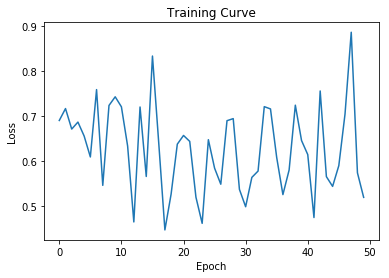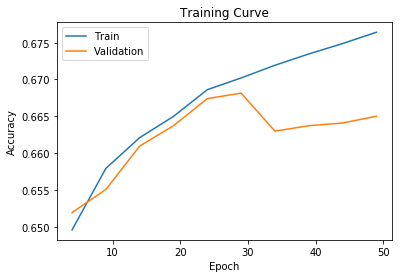Out:
0.6683259587020649

We can try a smaller network:

In :
mymodel = nn.Linear(50, 2)

Epoch 5; Loss 0.780455; Train Acc 0.570893; Val Acc 0.566003
Epoch 10; Loss 0.677516; Train Acc 0.598058; Val Acc 0.593473
Epoch 15; Loss 0.772151; Train Acc 0.619384; Val Acc 0.608591
Epoch 20; Loss 0.505169; Train Acc 0.631000; Val Acc 0.628319
Epoch 25; Loss 0.679968; Train Acc 0.639297; Val Acc 0.637537
Epoch 30; Loss 0.569500; Train Acc 0.643783; Val Acc 0.646386
Epoch 35; Loss 0.696912; Train Acc 0.650728; Val Acc 0.650258
Epoch 40; Loss 0.654828; Train Acc 0.653986; Val Acc 0.655605
Epoch 45; Loss 0.636120; Train Acc 0.656198; Val Acc 0.657817
Epoch 50; Loss 0.612439; Train Acc 0.658165; Val Acc 0.659661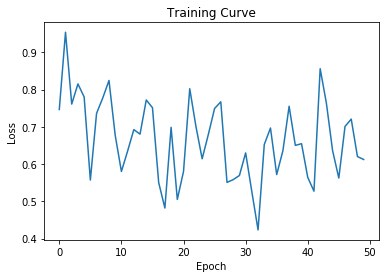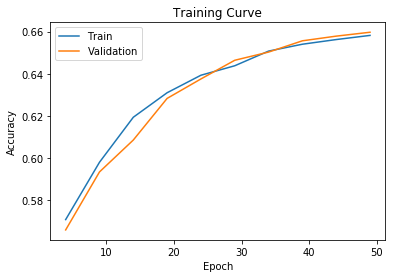Out:
0.6565265486725663

We can also try using a larger dimensional embedding:

In :
glove = torchtext.vocab.GloVe(name="6B", dim=100, max_vectors=20000)
train, valid, test = get_tweet_vectors(glove)

mymodel = nn.Sequential(nn.Linear(100, 45), nn.ReLU(), nn.Linear(45, 2))

Epoch 5; Loss 0.613013; Train Acc 0.641448; Val Acc 0.645096
Epoch 10; Loss 0.652138; Train Acc 0.667814; Val Acc 0.665929
Epoch 15; Loss 0.495925; Train Acc 0.675496; Val Acc 0.670170
Epoch 20; Loss 0.537843; Train Acc 0.681519; Val Acc 0.673488
Epoch 25; Loss 0.547823; Train Acc 0.687481; Val Acc 0.678097
Epoch 30; Loss 0.620160; Train Acc 0.691599; Val Acc 0.679019
Epoch 35; Loss 0.504232; Train Acc 0.695040; Val Acc 0.677544
Epoch 40; Loss 0.576010; Train Acc 0.701002; Val Acc 0.680125
Epoch 45; Loss 0.499430; Train Acc 0.704505; Val Acc 0.678282
Epoch 50; Loss 0.675659; Train Acc 0.706902; Val Acc 0.685103
Epoch 55; Loss 0.347993; Train Acc 0.708991; Val Acc 0.681047
Epoch 60; Loss 0.527149; Train Acc 0.710344; Val Acc 0.682338
Epoch 65; Loss 0.829638; Train Acc 0.712433; Val Acc 0.681600
Epoch 70; Loss 0.589659; Train Acc 0.714031; Val Acc 0.681416
Epoch 75; Loss 0.615215; Train Acc 0.717043; Val Acc 0.680678
Epoch 80; Loss 0.595324; Train Acc 0.718702; Val Acc 0.681232
Epoch 85; Loss 0.471805; Train Acc 0.721160; Val Acc 0.681416
Epoch 90; Loss 0.421523; Train Acc 0.722574; Val Acc 0.683075
Epoch 95; Loss 0.706711; Train Acc 0.725340; Val Acc 0.681416
Epoch 100; Loss 0.425212; Train Acc 0.726200; Val Acc 0.682153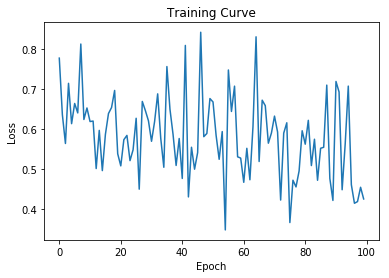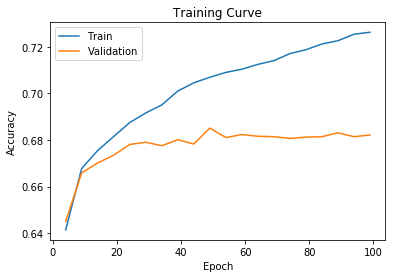Out:
0.68547197640118